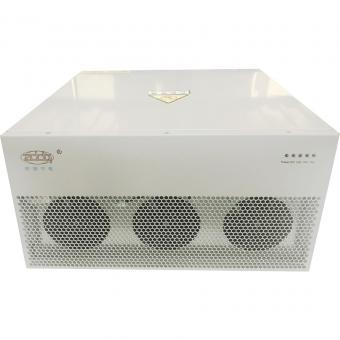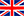English

###### Principle,function,structure,benefits of STATCOM
Mar 04,2020

STATCOM - Static Synchronous Compensator

It is a solid-state switching converter, capable of generating or absorbing independently controllable real and reactive power at its output terminals when it is fed from an energy source.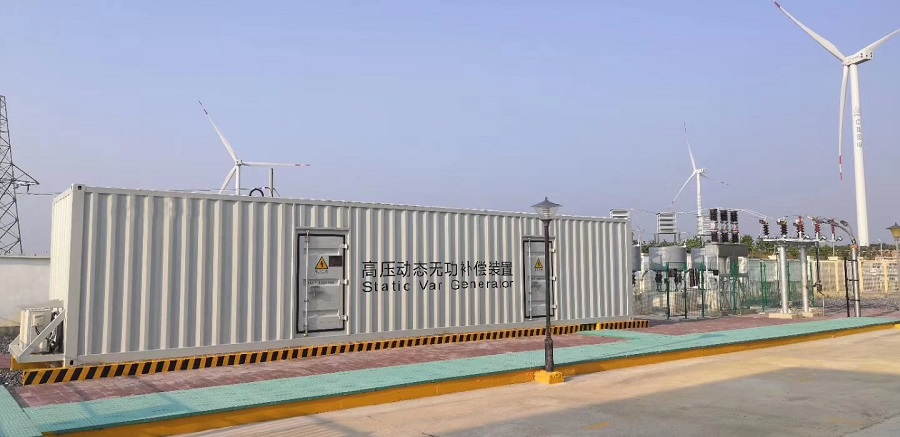STATCOM is considered as voltage-source converter that, from a given input of dc voltage, produces a set of 3-phase ac-output voltages, each in phase with and coupled to the corresponding ac system voltage through a relatively small reactance.

STATCOM can improve

1. the dynamic voltage control in transmission and distribution systems;
2. the power-oscillation damping in power-transmission systems;
3. the transient stability;
4. the voltage flicker control; and
5. the control of not only reactive power but also (if needed) active power in the connected line, requiring a dc energy source.

STATCOM structure

According to the application of the site, usually two types (outdoor and indoor) are installed

1. it occupies a small footprint,
2. it factory-built equipment, thereby reducing site work and commissioning time;
3. it uses encapsulated electronic converters, thereby minimizing its environmental impact.

A STATCOM is similar to an ideal synchronous machine, which generates a balanced set of three sinusoidal voltages—at the fundamental frequency—with controllable amplitude and phase angle.

When supplying/absorbing Reactive Power

 Reactive Power Generation Real Power Generation Magnitude Es>Et Generates reactive power Phase Es leads Et Generates real power Magnitude Es Absorbs reactive power Phase Es lags Et Absorbs real power

If the amplitude of the output voltage is increased above that of the utility bus voltage, Et, then a current flows through the reactance from the converter to the ac system and the converter generates capacitive- reactive power for the ac system.

If the amplitude of the output voltage is decreased below the utility bus voltage, then the current flows from the ac system to the converter and the converter absorbs inductive-reactive power from the ac system.

If the output voltage equals the ac system voltage, the reactive-power exchange becomes zero, in which case the STATCOM is said to be in a floating state. Refer to figure 1.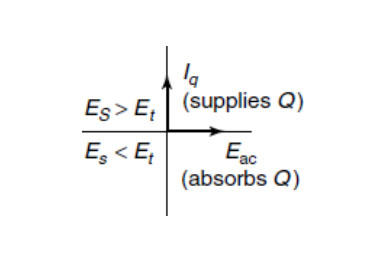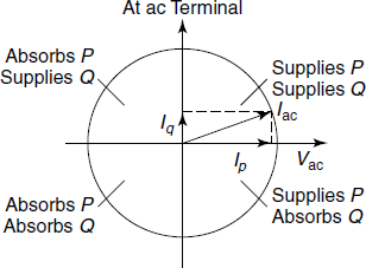Figure 1 Reactive Power Generation Figure 2  Real Power Generation

In reactive power generation, the real power provided by the dc source as input to the converter must be zero. The primary need for the capacitor is to provide a circulating-current path as well as a voltage source.

In practice, the semiconductor switches of the converter are not lossless, so the energy stored in the dc capacitor is eventually used to meet the internal losses of the converter, and the dc capacitor voltage diminishes.

Hence by making the output voltages of the converter lag behind the ac-system voltages by a small angle (usually in the 0.1–0.2 degree range), the converter absorbs a small amount of real power from  the ac system to meet its internal losses and keep the capacitor voltage at the desired level.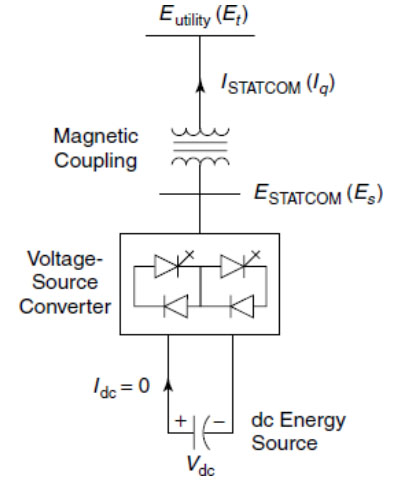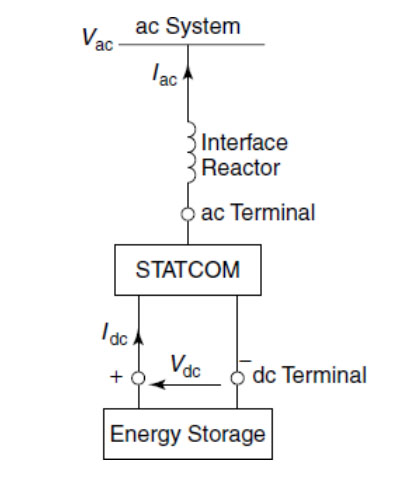Figure 3 STATCOM power circuit Figure 4 STATCOM power circuit with energy storage

When supplying/absorbing Real Power

Adjusting the phase shift between the converter-output voltage and the ac system voltage can similarly control real-power exchange between the converter and the ac system.

If the converter-output voltage is made to lead the ac-system voltage, then the converter can supply real power to the ac system from its dc energy storage.

If its voltage lags behind the ac-system voltage, then the it absorb real power from the ac system for the dc system. Refer to Figure 2.

V-I Characteristic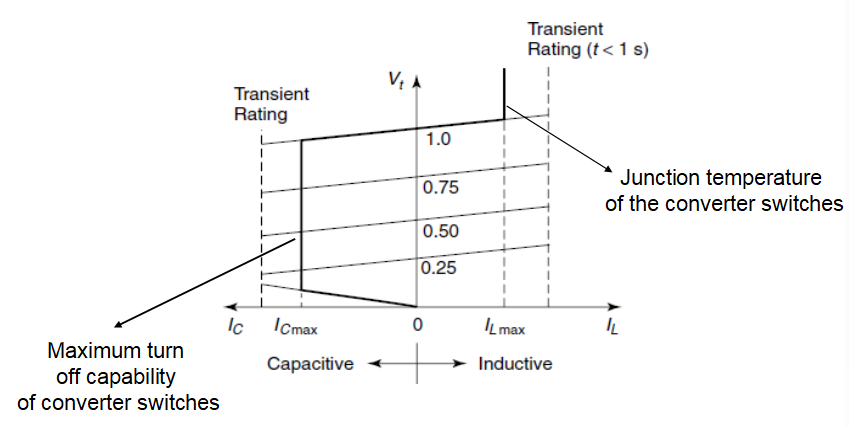Figure 5 V-I Characteristic of STATCOM

STATCOM can supply both the capacitive and the inductive compensation and is able to independently control its output current over the rated maximum capacitive or inductive range irrespective of the amount of ac-system voltage. That is, the STATCOM can provide full capacitive-reactive power at any system voltage—even as low as 0.15 pu.

The characteristic of a STATCOM reveals another strength of this technology:

1. It is capable of yielding the full output of capacitive generation almost independently of the system voltage.
2. Hence it supports the system voltage during and after faults where voltage collapse would otherwise be a limiting factor.

The maximum attainable transient over-current in the capacitive region is determined by the maximum current turn-off capability of the converter switches. In the inductive region, the converter switches are naturally commutate.

Blog
Hot Products
•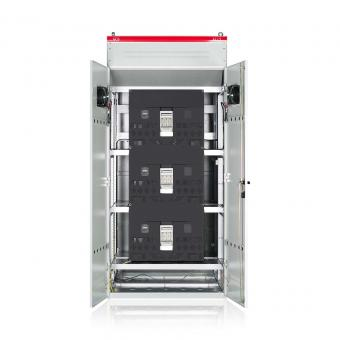#### Capacitor banks and harmonic filters. Low voltage

•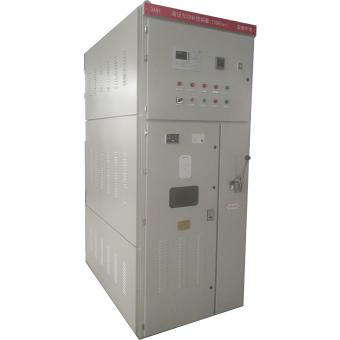#### 3.3KV Local reactive power compensation for motors,Arc Furnace

•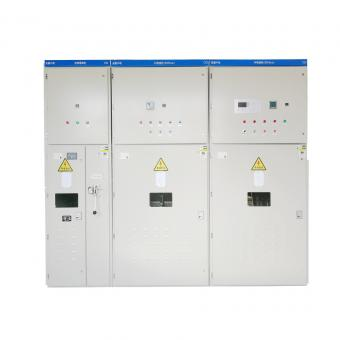#### Medium voltage Automatic Capacitor banks with harmonic filters

•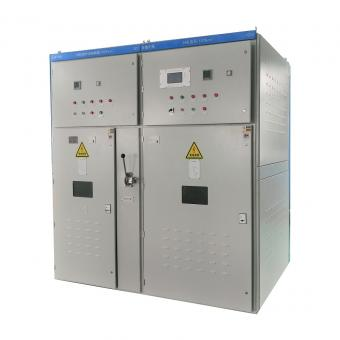#### High voltage Automatic Power Factor improvement plant for Motors VFDs

•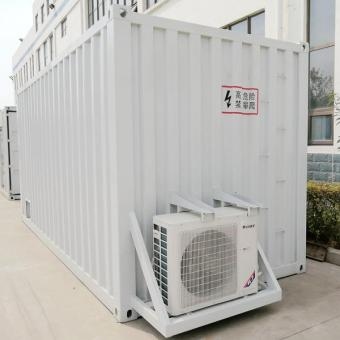#### High voltage static var generator HV SVG Statcom outdoor container

•#### Medium voltage outdoor Static Synchronous Compensator(STATCOM)

•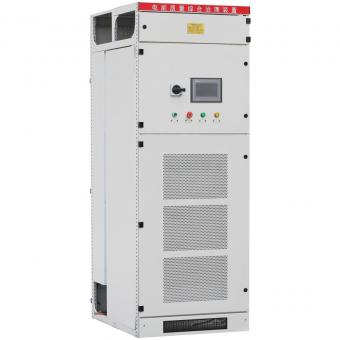•